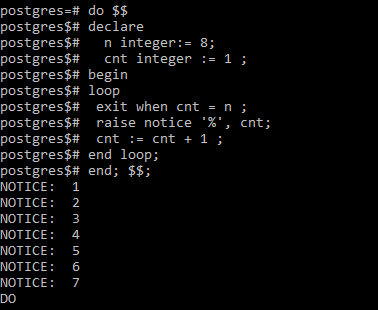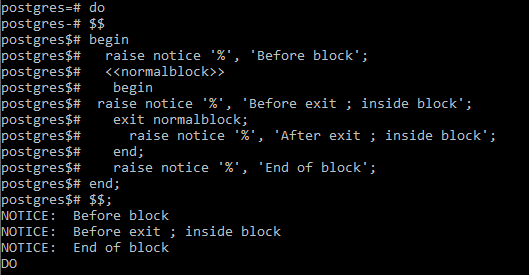Related Articles

# PostgreSQL – Exit

• Last Updated : 08 Feb, 2021

In PostgreSQL, The EXIT statement is used to terminate all types of loops like unconditional loops, a while loop, or a for loop or terminate a block of code specified by the begin..end keywords.

### Using EXIT for loops

We can use the exit to terminate looping statements using the following syntax:

#### Syntax:

`exit [label] [when condition]`

If we analyze the above syntax:

• Label: The label is used to signify the loop which we want to exit. It is often used in the case of nested looping. If a label is not present, the current loop is terminated.

• Condition: The condition is a simple boolean expression that determines when we want to terminate the loop. When the value of the boolean expression becomes true, the loop is terminated.

Both of the above are optional. We can use exit with a condition like:

`exit when cnt < 5;`

Without using the condition in exit, we can rewrite the same code using the IF statement as:

```if cnt < 5 then
exit;
end if;```

#### Example:

Suppose we a have loop that is used to print all numbers from 1 to 10. We can use the EXIT statement in the following manner to limit printing the numbers up to 7 only.

```do \$\$
declare
n integer:= 8;
cnt integer := 1 ;
begin
loop
exit when cnt = n ;
raise notice '%', cnt;
cnt := cnt + 1 ;
end loop;
end; \$\$;```

Output:In the above example, we terminate our loop as soon as the value of our cnt variable reaches n(here 8) and thus, only values up to 7 are printed.

### Using EXIT to exit a block

We can then the exit statement to terminate a block of code specified by the begin..end keywords. In this case, the exit directly passes the flow of the program to after the end keyword, thus ending the current block.

#### Syntax:

```<<block_label>>
BEGIN
Statements
EXIT [block_label] [WHEN condition];
Statements
END block_label;```

Using this syntax, we can terminate the block of code prematurely, thus preventing the statements after the exit to be run.

#### Example:

The following example shows how we can use EXIT to exit a block.

```do
\$\$
begin
raise notice '%', 'Before block';
<<normalblock>>
begin
raise notice '%', 'Before exit ; inside block';
exit normalblock;
raise notice '%', 'After exit ; inside block';
end;
raise notice '%', 'End of block';
end;
\$\$;```

Output:In the above example, the statement after exit was not printed as the block was terminated using EXIT before the statement. Thus inside the block, only statements before EXIT were executed and after that, the flow simply passes after the block ended.

My Personal Notes arrow_drop_up